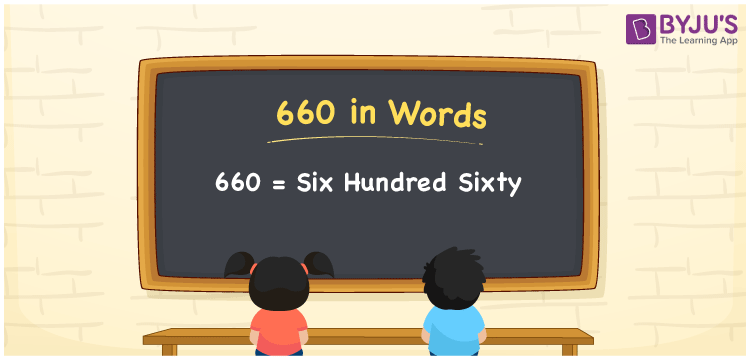# 660 in words

660 in words is written as Six Hundred and Sixty. 660 represents the count or value. The article on Counting Numbers can give you an idea about count or counting. The number 660 is used in expressions that relate to money, days, distance, length, weight and more. Let us consider an example for 660. “A Flight can carry upto Six Hundred and Sixty passengers.” Another example, “Six Hundred and Sixty days is less than two years.”

 660 in words Six Hundred and Sixty Six Hundred and Sixty in Numbers 660

## 660 in English Words## How to Write 660 in Words?

We can convert 660 to words using a place value chart. The number 660 has 3 digits, so let’s make a chart that shows the place value up to 3 digits.

 Hundreds Tens Ones 6 6 0

Thus, we can write the expanded form as:

6 × Hundred + 6 × Ten + 0 × One

= 6 × 100 + 6 × 10 + 0 × 1

= 660

= Six Hundred and Sixty.

660 is the natural number that is succeeded by 659 and preceded by 661.

660 in words – Six Hundred and Sixty.

Is 660 an odd number? – No.

Is 660 an even number? – Yes.

Is 660 a perfect square number? – No.

Is 660 a perfect cube number? – No.

Is 660 a prime number? – No.

Is 660 a composite number? – Yes.

## Solved Example

1. Write the number 660 in expanded form

Solution: 6 × 100 + 6 × 10 + 0 × 1

We can write 660 = 600 + 60 + 0

= 6 × 100 + 6 × 10 + 0 × 1

## Frequently Asked Questions on 660 in words

Q1

### How to write 660 in words?

660 in words is written as Six hundred and Sixty.
Q2

### Is 660 divisible by 2?

Yes. 660 is divisible by 2.
Q3

### Is 660 a perfect square number?

No. 660 is not a perfect square number.